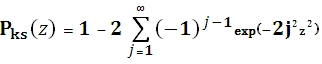# SQL Server KS upper cumulative distribution function

KSQINV

Updated: 29 June 2012

Use KSQINV to calculate the inverse of the complementary Kolmogorov-Smirnov (KS) cumulative distribution function, Qks = 1 - Pks. The equation for the cumulative distribution function is:Syntax
SELECT [wctStatistics].[wct].[KSQINV] (
<@Q, float,>)
Arguments
@Q
is the value at which to evaluate the function. @Q is an expression of type float or of a type that can be implicitly converted to float
Return Types
Float
Remarks
·         Qz must greater than zero and less than or equal to one
·         Qks(0) = 1
·         Qks(8) = 0
Examples
SELECT wct.KSQINV(0.269999671677355) as KSQINV
This produces the following result.
KSQINV
----------------------
1

### SupportCopyright 2008-2023 Westclintech LLC         Privacy Policy        Terms of Service